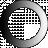Loading...

# American Dictionary of the English Language

Dictionary Search

### Proportional

PROPORTIONAL, adjective Having a due comparative relation; being in suitable proportion or degree; as, the parts of an edifice are proportional In pharmacy, medicines are compounded of certain proportional quantities of ingredients. The velocity of a moving body is proportional to the impelling force, when the quantity of matter is given; its momentum is proportional to the quantity of matter it contains, when its velocity is given.

PROPORTIONAL, in chimistry, a term employed in the theory of definite proportions, to denote the same as the weight of an atom or a prime. [See Prime.]

PROPORTIONALs, in geometry, are quantities, either linear or numeral, which bear the same ratio or relation to each other.